×

Search anything:

# Early Exit in ML models

#### Machine Learning (ML)Get this book -> Problems on Array: For Interviews and Competitive Programming

In this article, we will see what are the benefits of adding early exits to the neural network models and how it increases the performance of the model and overcomes various limitations present in the model.

1. Introduction to Problems in Neural Network
2. What exactly are these early exits?
3. Selecting when and where to add these Early exits
4. Adding an appropriate interference mechanism
5. Properties of the Neural Networks with multiple early exits?

Let us get started with this.

# Introduction to Problems in Neural Network

Usually, the Neural Networks are pretty deep with multiple layers. And it has its benefits such as modularity and compositionality of the network. But having deep layers also has its drawbacks like overfitting of data, difficulties in parallelizing the network due to the presence of gradient locking problem, and so on.

In some models the predictions that are correct in the early stage become incorrect towards the end of the network, this phenomenon is called Overthinking. One of the best ways to tackle such problems is the use of multiple early exits.

So we will see how these drawbacks or problems can be compromised by the use of early exits.

# What exactly are these early exits?

It is exactly what it sounds like, an early exit is nothing but an intermediate output in the network, which takes the calculations of the network in the early stage of the network i.e. before the network ends, and generates a prediction/result from those of calculation.

Here we will be discussing the use of auxiliary classifiers as early exits in the architectures. Usually, the models have one or two of these classifiers in them to simplify gradient propagation.

And we will also see how the use of multiple early exits is useful to tackle the conventional problems and we will look into the other benefits it provides to the network like regularization of the computational cost, improving the efficiency of the inference phase, just to name a few.

# Neural Networks with early exits

Consider a neural network made up of L layers that takes an input x and gives an output y. Here, the input x can be an image, a graph, a video, or any other structured data type.

And each layer or block of the neural network is a sequence of operations or calculations carried out on the input data and it is represented by $$f_i$$ and the output of the $$f_i$$ th layer is denoted by $$h_i$$

We begin by considering various midpoints to add our early exits into the model. At these points, we take the output of the layer at that point and feed it into an auxiliary classifier to obtain the intermediate prediction/result.

It can be more accurately represented with this equation.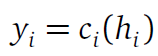Here,

• $$h_i$$ is the output from the layer at the midpoint
• $$c_i$$ represents the i th Auxiliary Classifier typically made up of 1 or 2 layers
• And $$f_i$$ is the intermediate prediction (the ith early exit)

Visually the addition of an early exit looks like this in a model: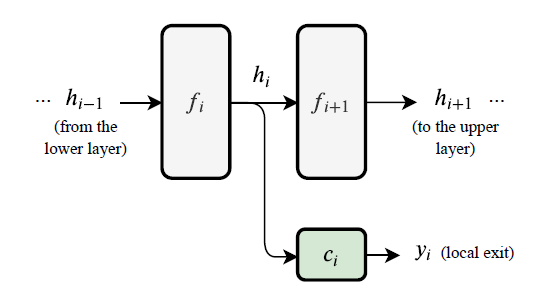By running a neural network with multiple such early exits we obtain a sequence of prediction $$y_i$$ that are more refined and accurate predictions at each level than the previous one of the network.

Let's look at more details about how these multiple early exits are attributed to a neural network.

# Selecting when and where to add these Early exits

The selection of points where the early exits are going to be assigned is also very important because the addition of early exits must speed up the inference phase and a simple way to do this is to calculate the relative computational cost of each layer in the network and add the early exits in suitable percentiles of the networks total cost.

And the type of auxiliary classifier must also be chosen with the structure of the network in mind, for example for the fully connected layers the use of simple Classifiers made up of one or two layers is enough, but in the case of CNNs, much complex classifiers are used.

And we must also be careful with the number of early exits. Consider this scenario, If our aim is just used to counter the problem of overfitting, it is sufficient enough to add just a few early exits. For example, the inception V1 model shown below has only two early exits placed roughly at the middle and before the end of the backbone network.

So we must use the required amount of early exits at the right place to get the most of the early exits.

## Training the Neural Networks with early exits

Some of the best approaches to train these neural networks with early exits are:

1. Joint Training Approach
2. Layer Wise Approach

Let us understand each approach.

1. Joint Training Approach

Here, a single optimization is carried out that comprises all intermediate exits result and output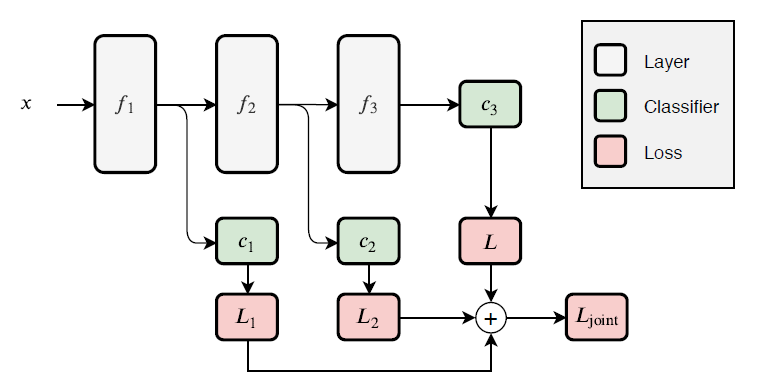2. Layer Wise Approach

In this approach, each auxiliary classifier is trained together with its backbone layers and then the classifier is frozen post training.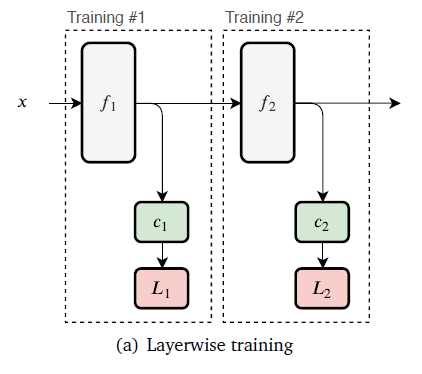# Adding an appropriate interference mechanism

After our neural network is trained, the next thing we must consider is the use of a suitable or apt interference mechanism or procedure. The interference mechanism is used to decide that after each early exit whether to exit the network or to continue processing to the next block in the network.

This addition of interference mechanism is trivial in some cases like the inception V1 model where the auxiliary classifiers are just used to improve the performance at the training phase, So the early exits are neglected and only the final prediction is considered.

The interference mechanism also seems to have other benefits like improvement in inference time, prevention of overfitting, and it can also tackle the problem of overthinking mentioned above.

# Properties of the Neural Networks with multiple early exits?

let's see what are the improvements in the neural network by the addition of these multiple early exits.

• The addition of early exits at the start of the architecture reduces the layer to layer weight computation and I/O costs, resulting in runtime and energy savings
• Early exit points provide regularization on the others, thus reduces the risk of overfitting and improves the test accuracy.
• Early exit points tend to provide additional and more intermediate gradient signals in back-propagation, resulting in more discriminative features in lower layers, thus improves accuracy.

One of the best examples of a model with multiple early exits is the BranchyNet, which has multiple branches from the backbone or the main branch, which results in its better performance and reduced inference time.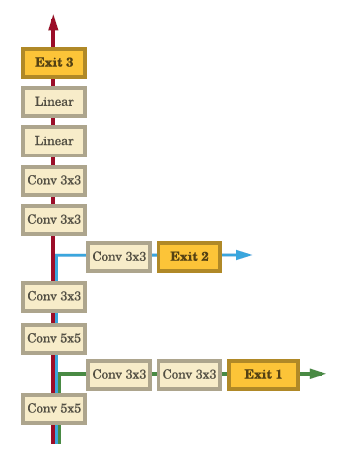# Conclusion

With this article at OpenGenus, you must have a strong idea of Early Exits in Machine Learning.

The addition of early exits to the neural systems has shown various improvements in the performance and the speed of the network. But still, the researchers believe that there are a lot more optimizations and advances that can be carried out on these neural networks.